# Analysis seminar

The Edinburgh Analysis Seminar is held in person at 2pm on Mondays in JCMB 5323. It is currently organised by Linhan Li and Leonardo Tolomeo

# Next talk

Thursday 1st June 2023, 4.p.m.

JCMB 5323

### Katarzyna Wyczesany

Abstract: In this talk, we will discuss order reversing quasi involutions, which are dualities on their image, and their properties. We prove that any order reversing quasi-involution is of a special form, which arose from the consideration of optimal transport problem with respect to costs that attain infinite values. We will discuss how this unified point of view on order reversing quasi-involutions helps to deeper the understanding of the underlying structures and principles. We will provide many examples and ways to construct new order reversing quasi-involutions from given ones. This talk is based on joint work with Shiri Artstein-Avidan and Shay Sadovsky.

# Full schedule

## Spring 2023

Monday, 16 Jan 2023

### Hiro Oh (University of Edinburgh)

Abstract:I will discuss well-posedness of the stochastic damped nonlinear wave equation (SdNLW) forced by a space-time white noise with the Gibbsian initial data. This problem is also known as the hyperbolic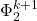-model since it corresponds to the so-called canonical stochastic quantization of the-measure. In this talk, our main goal is to study this problem on the plane.

I will first go over the well-posedness result on the two-dimensional torus studied by Gubinelli-Koch-Oh-Tolomeo (2022).  Then, by taking a large torus limit, I aim to construct invariant Gibbs dynamics for the hyperbolic-model on the plane.

In taking a large torus limit, I will discuss

(i) the construction of a-measure on the plane as a limit of the-measures on large tori. This is done by establishing coming-down-from-infinity for the associated stochastic nonlinear heat equation (SNLH) on the plane.

(ii) the construction of invariant Gibbs dynamics for the hyperbolic-model on the plane by taking a limit of the invariant Gibbs dynamics on large tori. Our strategy is inspired by a recent work by Oh-Okamoto-Tolomeo (2021) on the hyperbolic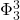-model on the three-dimensional torus, where we reduce the problem to studying convergence of the so-called enhanced Gibbs measures. By combining wave and heat analysis together with ideas from optimal transport theory, I establish convergence of the enhanced Gibbs measures.

This talk is based on a joint work with Leonardo Tolomeo (The University of Edinburgh), Yuzhao Wang (University of Birmingham), and Guangqu Zheng (University of Liverpool).

Monday, 30 Jan 2023

### Ruoyuan Liu (University of Edinburgh)

Abstract: In this talk, I will present results on local well-posedness of the nonlinear Schrödinger equation (NLS) with the quadratic nonlinearity |u|^2, posed on the two-dimensional torus, from both determinstic and probabilistic points of view.

For the deterministic well-posedness, Bourgain (1993) proved local well-posedness of the quadratic NLS in H^s for any s > 0. In this talk, I will go over local well-posedness in L^2, thus resolving an open problem of 30 years since Bourgain (1993). In terms of ill-posedness in negative Sobolev spaces, this result is sharp. As a corollary, a multilinear version of the conjectural L^3-Strichartz estimate on the two-dimensional torus is obtained.

For the probabilistic well-posedness, I will talk about almost sure local well-posedness of the quadratic NLS with random initial data distributed according to a fractional derivative of the Gaussian free field, and also a certain probabilistic ill-posedness result when the initial data becomes very rough. In particular, this part of the talk shows that the prediction on the critical regularity made by the probabilistic scaling due to Deng, Nahmod, and Yue (2019) breaks down for this model.

The first part of the talk is based on a joint work with Tadahiro Oh (The University of Edinburgh).

Monday, 6 Feb 2023

### Emily Roff (University of Edinburgh)

Abstract: Magnitude is an isometric invariant of metric spaces, usually interpreted as assigning to each space a real-valued function on the positive half-line. Though its provenance is category-theoretic, the magnitude function of a compact metric space turns out to be a geometrically rich invariant, its asymptotics carrying subtle information about the intrinsic volumes of a space and the Willmore energy.

In recent years there have been rapid advances in our understanding of the magnitude function, particularly for submanifolds of Euclidean space—yet, many interesting questions still stand open. This talk will introduce the theory and survey recent results due to Gimperlein, Goffeng, Louca and Meckes. I will also describe aspects of my own work, joint with Leinster and extending results due to Leinster and Meckes, which link the study of magnitude to problems in the quantification of ecological diversity. Finally, I will sketch the connection to magnitude homology, an algebraic invariant designed to categorify’ the magnitude function. Throughout, I will foreground open questions of an analytic nature.

Monday, 13 Feb 2023

### Andrew Rout (University of Warwick)

Abstract: Gibbs measures for the nonlinear Schrödinger equation (NLS) are important objects used to study low-regularity almost sure well-posedness. On the other hand, the NLS can be viewed as a limit of many-body quantum mechanics. In this talk we describe the derivation of Gibbs measures from their many-body quantum analogues.In particular, we consider dimension d=1 with a non-positive interaction potential. The proof is based off a perturbative expansion of the Hamiltonian and a diagrammatic representation of the interaction.

This is based on joint work with Vedran Sohinger.

Monday, 20 Feb 2023

### Valentina Ciccone (Universität Bonn)

Abstract:In this talk, we discuss a sharp Fourier extension inequality on the circle in the Stein-Tomas endpoint case for functions whose spectrum satisfies a certain arithmetic constraint. Such arithmetic constraint corresponds to a generalization of the notion of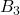-set.

This talk is based on a joint work with Felipe Gonçalves.

Monday, 6 March 2023

### Spyridon Kakaroumpas (University of Würzburg)

Abstract:

Let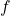be a finite Blaschke product on the unit disk with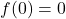that is not a rotation. Motivated by earlier results of M.~Weiss on lacunary power series, J.~J.~Donaire and A.~Nicolau proved that if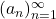is a sequence of complex numbers such that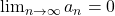and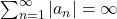, then for all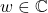, there exists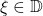such that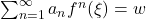, where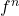denotes the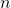-th iterate of(i.~e.~its composition with itselftimes).
In this talk we discuss an extension of this result, which shows that under the same conditions on the coefficients, the set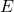of allsuch that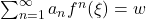has Hausdorff dimension 1. We use an iterative construction of appropriate Cantor-type sets, which depends on a careful analysis of the machinery developed by Donaire–Nicolau.
We also explore the optimality of our result under imposing more restrictive conditions on the coefficients. On the one hand, for any fixed gauge function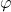that is “better” than any power function, we provide conditions which ensure that the sethas positive-Hausdorff content. On the other hand, we also provide conditions which ensure thathas zero-Hausdorff content, in the important special case that the Blaschke productis of the form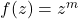.
This is joint work with O.~Soler i Gibert.

Monday, 13 March 2023

### Amlan Banaji (Loughborough University)

Abstract: Many fractals can be realised as the limit set of an iterated function system (IFS) of contracting maps. If the IFS consists of a countably infinite number of maps, then different notions of fractal dimension of the resulting limit set, such as Hausdorff, box and Assouad-type dimensions, can take different values, even if the contractions are assumed to be conformal and well-separated. We will explain what is known about these dimensions in this setting, and describe applications to parabolic Cantor sets, and to sets of numbers which have continued fraction expansions with restricted entries. This talk is based on joint work with Jonathan Fraser.

Monday, 27 March 2023

### Bruno Poggi Cevallos (Universitat Autònoma de Barcelona)

Abstract: On Corkscrew domains with Ahlfors-regular boundary, we prove the equivalence of the classically considered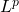-solvability of the (homogeneous) Dirichlet problem with the solvability of the inhomogeneous Poisson problem with interior data in an-Carleson space (with a natural bound on thenorm of the non-tangential maximal function of the solution), and we study several applications. Our main application is towards the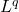Dirichlet-regularity problem for second-order elliptic operators satisfying the Dahlberg-Kenig-Pipher condition (this is, roughly speaking, a Carleson measure condition on the square of the gradient of the coefficients), in the geometric generality of bounded Corkscrew domains with uniformly rectifiable boundaries (although this problem had been open even in the unit ball). Other applications include: several new characterizations of the-solvability of the Dirichlet problem, new non-tangential maximal function estimates for the Green’s function, a new local T1-type theorem for thesolvability of the Dirichlet problem, new estimates for eigenfunctions, free boundary theorems, and a bridge to the theory of the Filoche-Mayboroda landscape function (also known as torsion function). This is joint work with Mihalis Mourgoglou and Xavier Tolsa.

Friday, 31 March 2023, 14:00 – 17:30, Appleton Tower Lecture Theatre 3

### Maxwell Mini-Symposium in Harmonic Analysis and PDE

https://www.maths.ed.ac.uk/~toh/Files/2023MI/MI03_2023.html

### Maciej Zworski (UC Berkeley, USA)

Title: Propagation of Smallness, Control and Stabilisation

Abstract: Control and stabilization are now well understood when controls or damping act on open sets. When they act on measurable sets only, much remains to be understood. In this talk I will present results in this direction. In most cases (but not always) the results I will present will rely on the quantitative results of Logunov/Malinnikova for harmonic functions that can be translated into control or stabilisation results for heat, waves or Schrodinger equations. Most of the results presented in the talk are in collaboration with I. Moyano (Univ. Nice).

Title: Derivation of nonlinear Gibbs measures from many-body quantum mechanics

Abstract: In this talk, I will define and discuss some probability measures in infinite dimensions, which play an important role in (S)PDE, in Quantum Field Theory and for the description of Bose-Einstein condensates. Those are Gibbs measures associated with nonlinear Schrodinger-type energies. In dimensions larger than or equal to 2, the measures concentrate on distributions, and they need to be properly renormalized. After presenting the Gibbs measures, I will explain how to derive them from many-body quantum mechanics. Joint works with Phan Thanh Nam (Munich) and Nicolas Rougerie (Lyon).

Title: Mathematics of magic angles

Abstract: Magic angles are a hot topic in condensed matter physics: when two sheets of graphene are twisted by those angles the resulting material is superconducting. I will present a very simple operator whose spectral properties are thought to determine which angles are magical. It comes from a 2019 PR Letter by Tarnopolsky–Kruchkov–Vishwanath. The mathematics behind this is an elementary blend of representation theory (of the Heisenberg group in characteristic three), Jacobi theta functions and spectral instability of non-self-adjoint operators (involving Hormander’s bracket condition in a very simple setting). Recent mathematical progress also includes the proof of existence of generalized magic angles and computer assisted proofs of existence of real ones (Luskin–Watson, 2021). The results will be illustrated by colourful numerics which suggest many open problems (joint work with S Becker, M Embree, J Wittsten in 2020 and S Becker, T Humbert and M Hitrik in 2022).

Monday, 3 April 2023, 2.05pm

### Ryan Unger (Princeton University)

Abstract: In this talk I will present a rigorous construction of examples of black hole formation which are exactly isometric to extremal Reissner–Nordström after finite time. In particular, our result can be viewed as a definitive disproof of the “third law of black hole thermodynamics.” This is joint work with Christoph Kehle.

Monday, 3 April 2023, 3.00pm  JCMB_Lecture Theatre B

### Simão Correia (Instituto Superior Técnico)

Abstract:  Self-similar solutions for the modified Korteweg-de Vries equation areof both physical and mathematical interest. On the physical side, amongseveral applications, they model the formation of sharp corners inplanar vortex patches. Mathematically, they describe the asymptoticbehavior of solutions for large times and present a blow-up behavior atthe initial time. Due to their scaling invariance, these solutionsdisplay several critical features (time decay, spatial decay andregularity), which means that the existing theory is not applicable. Inthis talk, I will show that one can define the flow around thesesolutions in order to analyze both the long-time and the blow-upasymptotics. Afterwards, I will present a stability result at theblow-up time and discuss future developments. This is joint work with R.Côte and L. Vega.

Friday, 21 April 2023  from 2.00 p.m, Saturday 22nd April 2023 from 9.30.a.m, George Square area

Abstract: TBA

Abstract: TBA

## Summer 2023

Monday, 24 April 2023

### Antoine Julia (Université Paris-Saclay)

Abstract: I will present three definitions of harmonic measure in plane domains, and discuss the relationship between the regularity of the harmonic measure and that of the domain. In particular I will explain how one can construct an irregular domain (the complement of a certain Cantor set) for which the harmonic measure is regular. This is based on joint work with Guy David and Cole Jeznach.

Monday, 1 May 2023

### Joseph Feneuil (Australian National University)

Abstract:

The past 10 years have seen considerable achievements at the intersection of harmonic analysis, PDE, and geometric measure theory. One now better understands the relationship between the geometry of the boundary of a domain and the regularity of harmonic/elliptic solutions inside the domain. For instance, it was proved that the uniform rectifiability (UR) of a codimension 1 set is characterized by the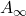-absolute continuity of its harmonic measure with respect to the surface measure – or equivalently the solvability of aDirichlet problem in the complement.
In this talk, I will show that another characterization of UR sets of codimension 1 can be obtained by comparing Green function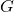with some regularized version of the distance to the boundary. Moreover, I will obtain a characterization of any UR set of any codimension by an estimate on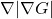. Those are joint works with Guy David, Linhan Li, and Svitlana Mayboroda.

Thursday 1st June 2023, 4.p.m.

JCMB 5323

### Katarzyna Wyczesany

Abstract: In this talk, we will discuss order reversing quasi involutions, which are dualities on their image, and their properties. We prove that any order reversing quasi-involution is of a special form, which arose from the consideration of optimal transport problem with respect to costs that attain infinite values. We will discuss how this unified point of view on order reversing quasi-involutions helps to deeper the understanding of the underlying structures and principles. We will provide many examples and ways to construct new order reversing quasi-involutions from given ones. This talk is based on joint work with Shiri Artstein-Avidan and Shay Sadovsky.

# Upcoming Semester

## Autumn 2023

Monday, 18 September  2023

### Demi Allen (University of Exeter)

Abstract: TBA

Monday, 25 September  2023

Abstract: TBA

# Previous Semester

## Autumn 2022

Monday, 26 September 2022

### Arpad Benyi (Western Washington University)

Abstract: We will begin with an outline of the general setting and the history of several operators of interest in harmonic analysis. Then, we will discuss the continuity of some operators stemming from a maximally modulated bilinear Hilbert transform along a cubic curve.

Monday, 03 October 2022

### Younes Zine (University of Edinburgh)

Abstract: Over the last twenty years there has been significant progress in the well-posedness study of singular stochastic PDEs in both parabolic and dispersive settings. In this talk, I will discuss some convergence problems for singular stochastic nonlinear PDEs. In a seminal work, Da Prato and Debussche (2003) established well-posedness of the stochastic quantization equation, also known as the parabolic Φk+12-model in the two-dimensional case. More recently, Gubinelli, Koch, Oh, and Tolomeo proved the corresponding well-posedness for the canonical stochastic quantization equation, also known as the hyperbolic Φk+12-model in the two-dimensional case. In the first part of this talk, I will describe convergence of the hyperbolic Φk+12-model to the parabolic Φk+12-model. In the dispersive setting, Bourgain (1996) established well-posedness for the dispersive Φ42-model (=deterministic cubic nonlinear Schrödinger equation) on the two-dimensional torus with Gibbsian initial data. In the second part of the talk, I will discuss the convergence of the stochastic complex Ginzburg-Landau equation (= complex-valued version of the parabolic Φ42-model) to the dispersive Φ42-model at statistical equilibrium.

Monday, 10 October 2022

### Itamar Oliveira (Université de Nantes)

Abstract: In this talk we will propose a new way of using lower dimensional information to obtain higher dimensional ones in the context of the Fourier restriction/extension problem. We will focus on the simplest multilinear case, which is the bilinear problem for functions in 2 variables supported in disjoint caps of the paraboloid; in this setting, we will recover Tao’s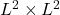into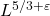bound assuming that one of the functions is a tensor. Broadly speaking, the proof depends on carefully combining a vector-valued version of the one-dimensional analog result (into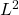) and curvature considerations. If time allows, we will talk about the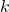-linear problem for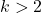, where the method achieves the conjectured exponent up to the endpoint assuming one tensor structure, but now under weak transversality rather than classical transversality.

Monday, 17 October 2022

### Bodan Arsovski (UCL)

Abstract: In this talk, we prove the natural analogue of the Kakeya conjecture in the alternative ambient space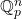, and we discuss some philosophical similarities and differences between this variant of the Kakeya conjecture and the classical Kakeya conjecture in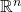.

Monday, 24 October 2022

### Jinyeop Lee (LMU Munich)

Abstract: In this talk, we derive effective dynamics governed by PDEs (nonlinear Schrodinger equation, Vlasov-Poisson equation) from the N-body Schrödinger equation with interactions in the large N limit. For the derivation, we first visit quantum mechanics. Then, using the knowledge of many-body quantum mechanics given in the talk and the suitable conditions for each situation, we derive PDEs describing the effective motion of the system.

Friday, 4 November 14:00 — 17:40, Newhaven Lecture Theatre (13-15 South College Street)

### Matteo Capoferri (HW), Jan Sbierski (UoE), Leonardo Tolomeo (UoE), Jiawei Li (UoE)

14:00-14:40 Matteo Capoferri: Spectral theory of systems of PDEs

14:50-15:30 Jan Sbierski: On the uniqueness problem for extensions of Lorentzian manifolds

15:35-16:05 Coffee break

16:05-16:45 Leonardo Tolomeo: Phase transitions of the focusing Φ^p_1 measures

16:55-17:35 Jiawei Li: On distributions of random fields in turbulent flows

Matteo Capoferri:

Title: Spectral theory of systems of PDEs

Abstract: I will present a new approach to the spectral theory of systems of PDEs on closed manifolds, developed in a series of recent papers by Dmitri Vassiliev (UCL) and myself, based on the use of pseudodifferential projections. Emphasis will be placed on ideas and motivation; the talk will include a brief historical overview of the development of the subject area.

Jan Sbierski:
Title: On the uniqueness problem for extensions of Lorentzian manifolds
Abstract: This talk discusses the problem under what conditions two extensions of a Lorentzian manifold have to be the same at the boundary. As will be explained, this question arises naturally in the analysis of Einstein’s equations of general relativity. After making precise the notion of two extensions agreeing at the boundary, we recall a classical example that shows that even under the assumption of analyticity of the extensions, uniqueness at the boundary is in general false — in stark contrast to the extension problem for functions on Euclidean space. We proceed by presenting a recent result that gives a necessary condition for two extensions with at least Lipschitz continuous metrics to agree at the boundary. Furthermore, we discuss the relation to a previous result by Chruściel and demonstrate a new non-uniqueness mechanism for extensions below Lipschitz regularity.
Leonardo Tolomeo:
Title: Phase transitions of the focusing Φ^p_1 measures

Abstract: We study the behaviour of invariant measures for the focusing nonlinear Schrödinger equation on the one-dimensional torus, initiated by Lebowitz, Rose, and Speer (1988). Because of the focusing nature of the measure, it is necessary to introduce a mass cutoff K, and restrict the measure to the set where the mass is smaller than K. We will show the following phase transitions:

– When K is smaller than a certain threshold, the measure is well defined, while for K bigger than the threshold, the measure becomes non-normalisable. We will also discuss what happens at the optimal threshold, and show that the measure is well defined in this case as well, solving a long-standing open problem. This is a joint work with T. Oh (University of Edinburgh) and P. Sosoe (Cornell University).

– When the size L of the torus is going to infinity, in the weakly non-linear regime, numeric simulations in the original paper by Lebowitz, Rose, and Speer (1988) suggest a phase transition depending on the temperature of the system. We show that this is indeed the case: if the temperature is high, the measure converges to a given gaussian measure. However, this convergence does not happen in the low-temperature regime, and instead the measure progressively concentrates around a single soliton. This is a joint work with H. Weber (University of Münster).
Jiawei Li:

Title: On distributions of random fields in turbulent flows

Abstract: In this talk, we are interested in the vorticity and velocity random fields of turbulent flows. I will introduce the evolution equations for probability density functions of these random fields under some conditions on the flow. I will also talk about several methods we used to solve these PDF PDEs numerically. Based on joint works with Zhongmin Qian and Mingrui Zhou.

Monday, 7 November 2022

### Natalia Jurga (University of St Andrews)

Abstract: A finite set of matrices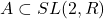acts on one-dimensional real projective space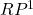through its linear action on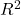. In this talk we will be interested in the limit set of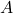: the smallest closed subset ofwhich contains all attracting fixed points of matrices belonging to the semigroup generated by. This talk will be a gentle introduction to various topics connected to the study of the geometry of these sets and we will discuss recent results concerning their Hausdorff dimension.

Monday, 14 November 2022

### Transversality in Harmonic Analysis and the Brascamp–Lieb Inequalities

Abstract: In modern harmonic analysis, there is a ubiquity of operators whose functional-analytic properties depend on the geometric properties of an underlying submanifold, such as Fourier extension operators and Radon-like transforms. In the analysis of the boundedness of such operators, it is often useful to employ a linear-to-multilinear reduction so that one may appeal to a multilinearised version of the linear estimate one would like to obtain. These multilinear estimates usually require that the submanifolds involved are uniformly transversal in some suitable sense, however, standard linear algebra tools are sometimes insufficient to capture an appropriately general notion of transversality for the estimates in which we are interested. Brascamp–Lieb inequalities offer a robust framework for understanding this higher-order notion transversality in a manner that is well-suited to applications in harmonic analysis. In my talk, I shall introduce the notion of a Brascamp–Lieb inequality, describe the broader role they play in the subject, and go on to discuss the topic of nonlinear Brascamp–Lieb inequalities, a recent variant that generalises this framework to the manifold setting.

Monday, 21 November 2022

### Stefan Czimek (Leipzig University)

Abstract: We present a new approach to the gluing problem in General Relativity, that is, the problem of matching two solutions of the Einstein equations along a spacelike or characteristic (null) hypersurface. In contrast to previous constructions, the new perspective actively utilizes the nonlinearity of the constraint equations. As a result, we are able to remove the 10-dimensional spaces of obstructions to gluing present in the literature. As application, we show that any asymptotically flat spacelike initial data set can be glued to Schwarzschild initial data of sufficiently large mass. This is joint work with I. Rodnianski.

Monday, 28 November 2022

### Lenny Neyt (Ghent University)

Abstract: We discuss necessary and sufficient conditions for when the surjectivity of a continuous linear operator between two locally convex spaces implies the surjectivity of the tensorized operator with some topological vector space. This is done via the presence of certain deep topological invariants on the kernel and the vector space, stemming from the application of tools from homological algebra into the field of functional analysis. As an application of these results we discuss the parameter dependence of solutions to partial differential equations and vector-valued extensions of Eidelheit sequences.

Monday, 5 December 2022, JCMB 5328, 2.05pm – 4.00pm

### Nicolas Camps

Abstract: Following the seminal works of Bourgain (1996), and Burq, Tzvetkov (2008), a statistical approach to nonlinear dispersive equations has developed in various contexts. One aspect of the theory is to generate full-measure sets of initial data that lead to strong solutions in certain regimes where instabilities are known to occur.

We discuss the probabilistic Cauchy theory in supercritical regimes for NLS in Euclidean space developed by Bényi, Oh, and Pocovnicu in 2015. In contrast, we proved with Louise Gassot that the pathological set —consisting of initial data that undergoes norm inflation— contains a dense G-delta set. This result is inspired by a construction of Lebeau (2001) and Sun-Tzvetkov (2019) for nonlinear wave equations.
The second part is devoted to the case of weakly dispersive equations in quasi-linear regimes, where the standard probabilistic Cauchy theory fails. We present recent developments based on a paracontrolled ansatz due to Bringmann (2019), which we exploit to prove almost-sure local well-posedness for a class of weakly dispersive equations such as the half-wave equation and the Szego equations. This last result is obtained in collaboration with Louise Gassot and Slim Ibrahim.

### Louise Gassot (University of Basel)

Abstract:We discuss the zero-dispersion limit for the Benjamin-Ono equation on the torus given a bell-shaped initial data. We prove that the solutions admit a weak limit as the dispersion parameter tends to zero, which is explicit and constructed from the Burgers’ equation. The approach relies on the complete integrability for the Benjamin-Ono equation from Gérard, Kappeler and Topalov, and also on the spectral study of the Lax operator associated to the initial data in the zero-dispersion limit.

## Summer 2022

Friday, 29 April 2022

### Ian Wood (University of Kent)

(Virtual Maxwell Analysis Seminar)

Abstract:

We determine and classify the spectrum of a non-selfadjoint operator pencil generated by the time-harmonic Maxwell problem with a nonlinear dependence on the frequency. More specifically, we consider one- and two-dimensional reductions for the case of two homogeneous materials joined at a planar interface. The dependence on the spectral parameter, i.e. the frequency, is in the dielectric function and we make no assumptions on its form. In order to allow also for non-conservative media, the dielectric function is allowed to be complex, yielding a non-selfadjoint problem. This is joint work with Malcolm Brown (Cardiff), Tomas Dohnal (Halle) and Michael Plum (Karlsruhe).

Friday, 13 May 2022

### Boris Thibert (Universite Grenoble Alpes)

(Virtual Maxwell Analysis Seminar)

Abstract:

Generated Jacobian Equations have been introduced in 2014 by Trudinger as a generalization of Monge-Ampère equations arising in optimal transport. They were originally motivated by inverse problems arising in non-imaging optics, where the goal is to design optical components, such as mirrors or lenses, that transfer a given source light to a prescribed target light.In this talk, I will present a Newton algorithm for solving Generated Jacobian equations in the semi-discrete setting, meaning that one of the measures involved in the problem is finitely supported and the other one is absolutely continuous. Then I will apply it to the design of different kinds of mirrors or lenses that allow to transfer any punctual or collimated source to any target. This work is in collaboration with Anatole Gallouet and Quentin Mérigot.

Wednesday, 13 July 2022

### Matteo Capoferri (Prifysgol Caerdydd)

Abstract:

The talk is concerned with the rigorous mathematical description of propagation and localisation of waves in a particular class of composite materials with random microscopic geometry, called micro-resonant (or high-contrast) random media: small inclusions of a “soft” material are randomly dispersed in a “stiff” matrix.  The highly contrasting physical properties of the two constituents,  combined with a particular scaling of the inclusions,  result in microscopic resonances, which manifest macroscopically by allowing propagation of waves in the material only within certain ranges of frequencies (band-gap spectrum).

High-contrast media with periodically distributed inclusions have been extensively studied and numerous results are available in the literature.  However,  their stochastic counterparts, which model more realistic scenarios and may exhibit localisation,  are far from being well understood from a mathematical viewpoint.  In my talk I will give an overview of existing results through the prism of stochastic homogenisation and spectral theory, and discuss recent advances and ongoing work.

Based on joint work with M. Cherdantsev and I. Velčić.

Monday, 8 August 2022

### Zoe Nieraeth (BCAM)

Abstract:

Vector-valued extensions of important operators in harmonic analysis have been actively studied in the past decades. A centerpoint of the theory is the result of Burkholder and Bourgain that the Hilbert transform extends to a bounded operator on L^p(R;X) if and only if the Banach space X has the so-called UMD property. In the specific case where X is a Banach function space, it is a deep result of Bourgain and Rubio de Francia that the UMD property is equivalent to the Hardy-Littlewood maximal operator having a bounded extension to both X and X’. In turn, this leads to powerful vector-valued extrapolation methods. In this talk I will place these ideas in the context of the more modern technique of domination by sparse forms. These forms are intimately related to Muckenhoupt weight classes and the multilsubinear Hardy-Littlewood maximal operator. Moreover, I will discuss some of work done in extending the UMD property to a multilinear setting. This talk is based on joint work with Emiel Lorist.

Wednesday, 10 August 2022

### Sam Collingbourne (University of Cambridge)

Abstract: I will discuss a direct rigorous mathematical proof of the Gregory–Laflamme instability for the 5D Schwarzschild black string. This is a mode instability at the level of the linearised vacuum Einstein equation. Under a choice of ansatz for the perturbation and a gauge choice, the linearised vacuum Einstein equation can be reduced to a Schrödinger eigenvalue equation to which an energy functional is assigned. It is then shown by direct variational methods that the lowest eigenfunction gives rise to an exponentially growing mode solution which has admissible behaviour at the future event horizon and spacelike infinity in harmonic/transverse-traceless gauge.

# Previous semesters (Virtual Maxwell Analysis Seminar)

## Spring 2022

Friday, 21st January 2022

### Odysseas Bakas (Basque Center for Applied Mathematics)

Abstract:

In 2011 Bonami, Grellier, and Ky proved that the product of a function in the Hardy space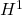and a function of bounded mean oscillation can be written, in the sense of distributions, as a sum of an integrable function and an element in an appropriate Hardy-Orlicz space. Moreover, they showed that the decomposition operators can be taken to be bilinear.

In this talk we present a dyadic analogue of the result of Bonami, Grellier, and Ky and we show how their theorem can be recovered from its dyadic counterparts. Related topics in one and several parameters will also be discussed.

This is joint work Sandra Pott, Salvador Rodríguez-López, and Alan Sola.

Friday, 4th February 2022

### Michael Tang (University of Edinburgh)

Abstract:

The multijoint problem is a discrete analogue to the multilinear Kakeya problem, and was solved by Zhang in 2016. More recently in 2020, Tidor, Yu and Zhao solved higher-dimensional generalisations of the multijoint problem. The multijoint and multilinear Kakeya problems can both be described by geometric multilinear inequalities for which there is a theory of duality, as established by Carbery, Hanninen and Valdimarsson. While the dual multilinear Kakeya problem is well understood, until now, the dual multijoint problem was not, despite the multijoint problem being solved by Zhang in 2016.

In this talk we formulate the multijoint problem as the boundedness of a multilinear operator and present a discrete analogue of a theorem due to Bourgain and Guth.

Friday, 11th February 2022

### Christoph Kehle (Insitute for Advanced Study)

Abstract:

The statement that general relativity is a deterministic theory finds its mathematical formulation in the celebrated ‘Strong Cosmic Censorship Conjecture’ due to Roger Penrose. I will present my recent results on the linear analog of this conjecture in the case of negative cosmological constant. It turns out that this is intimately tied to Diophantine properties of a suitable ratio of mass and angular momentum of the black hole and that the validity of the conjecture depends in an unexpected way on the notion of genericity imposed.

Friday, 25th February 2022

### João Pedro Ramos (ETH Zürich)

Abstract:

The celebrated isoperimetric inequality states that, for a measurable set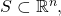the inequality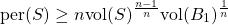holds, where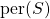denotes the perimeter (or surface area) of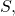and equality holds if and only if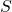is an euclidean ball. This result has many applications throughout analysis, but an interesting feature is that it can be obtained as a corollary of a more general inequality, the Brunn–Minkowski theorem: if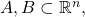define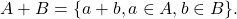Then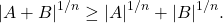Here, equality holds if and only ifand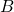are homothetic and convex. A question pertaining to both these results, that aims to exploit deeper features of the geometry behind them, is that of stability: ifis close to being optimal for the isoperimetric inequality, can we say thatis close to being a ball? Analogously, if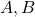are close to being optimal for Brunn–Minkowski, can we say they are close to being compact and convex?

These questions, as stand, have been answered only in very recent efforts by several mathematicians. In this talk, we shall outline these results, with focus on the following new result, obtained jointly with A. Figalli and K. Boroczky. If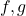are two non-negative measurable functions on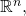and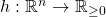is measurable such that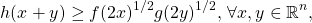then the Prekopa–Leindler inequality asserts that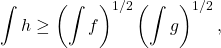where equality holds if and only if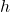is log-concave, andare homothetic’ to, in a suitable sense. We prove that, if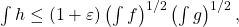then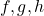are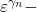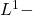close to being optimal. We will discuss the general idea for the proof and, time-allowing, discuss on a conjectured sharper version.

Friday, 4th March 2022

### Priscila Leal da Silva (Loughborough)

Abstract:

Since the discovery of the Camassa-Holm equation as an integrable peakon-equation, intense research has been dedicated to its generalisations of it and to the study of their solutions. Of particular interest in this talk is the rotation-Camassa-Holm equation (rCH), a nonlocally evolutive equation that takes into consideration the Coriolis force, which is typically a manifestation of rotation when Newton’s laws are applied to model physical phenomena on Earth’s surface. In this talk we present conditions for the existence of traveling wave solutions and show that, given an initial data in Sobolev spaces, uniqueness of local solutions is achieved. Moreover, assuming a strong condition on the McKean quantity, the solutions can be extended globally. Extensions of these results are presented, as well as further conjectures.

Friday, 11th March 2022

### Julian Braun (Heriot–Watt)

Abstract:

Lattice defects in crystalline materials (for example, a screw dislocations or an interstitial) create long-range elastic fields. In this presentation I will show how to rigorously derive a far-field expansion up to arbitrary high order. The expansion is computable and leaves only a fast decaying remainder describing the defect core structure. As an application I will also show how to use this expansion for high accuracy defect computations.

Friday, 18th March 2022

### Louise Gassot (ICERM  / University of Basel)

Abstract:

We discuss the zero-dispersion limit for the Benjamin-Ono equation on the torus given a single well initial data. We prove that there exist approximate initial data converging to the initial data, such that the corresponding solutions admit a weak limit as the dispersion parameter tends to zero. The weak limit is expressed in terms of the multivalued solution of the inviscid Burgers equation obtained by the method of characteristics. We construct our approximation by using the Birkhoff coordinates of the initial data, introduced by Gérard, Kappeler and Topalov.

Friday, 1st April 2022

### Annalisa Massaccesi (Università di Padova)

Abstract:

Abstract: In this seminar I will review the theory of flat G-chains, as they were introduced by H. W. Fleming in 1966, and currents with coefficients in groups. One of the most recent developments of the theory concerns its application to the Steiner tree problem and other minimal network problems which are related with a Eulerian formulation of the branched optimal transport. Starting from a 2016 paper by A. Marchese and myself, I will show how these problems and their variants are equivalent to a mass-minimization problem in the framework of currents with coefficients in a (suitably chosen) normed group.

## Autumn 2021

Friday, 1st October 2021

### Matias Ruiz (University of Edinburgh)

Abstract:

In the last couple of decades, the field of nanophotonics, i.e. the study of light-matter interactions at the nanoscale, has gained enormous importance in different disciplines of science and engineering. A large portion of this field concerns the study of electromagnetic resonances in nanoparticles which are, in turn, the building blocks of many applications in energy, healthcare, material science, to name a few.

The description of these resonances relies on interesting math problems with strong connections to PDE spectral problems, both self-adjoint and non-self-adjoint. As such, there is a growing interest from the math world in tackling some of the mathematical issues arising in nanophotonics. For instance, a canonical problem is the Laplace transmission problem showing a sign-change in the PDE coefficients, whose solutions can be understood in terms of the spectral properties of the Neumann-Poincare operator (and/or the so-called plasmonic eigenvalue problem).

In this applied analysis talk I will introduce some of the mathematically interesting problems arising in nanophotonics and discuss recent analytical results in the analysis of resonances in metallic nanoparticles (also known as plasmonic resonances), which include the use of layer potential techniques and asymptotic analysis.

Friday, 8th October 2021

### Jean-Claude Cuenin (Loughborough University)

Abstract:

I will give a survey of how methods from harmonic analysis, particularly those related to Fourier restriction theory, can be used in spectral and scattering theory. Applications include eigenvalue estimates for Schrödinger operators with complex potentials and almost sure scattering for random lattice Schrödinger operators in 3d with slowly decaying potential.

Friday, 15th October 2021

### Simon Myerson (University of Warwick)

Abstract:

Given the Laplace(-Beltrami) operator on a Riemannian manifold, consider the spectral projector on (generalized) eigenfunctions whose eigenvalue lies in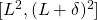– where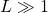and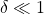. We ask the question of optimaltobounds for this operator. I will present new results in this direction for the Euclidean torus (joint with Pierre Germain).

Friday, 22nd October 2021

### Guido De Philippis (CIMS)

Abstract:

Michael Simon inequality is a fundamental tool in  geometric analysis and geometric measure theory.  Its extension to anisotropic integrands will allow to extend to anisotropic integrands a series of results which are currently known only for the area functional.

In this talk I will present an anistropic  version of the Michael-Simon inequality, for for two-dimensional varifolds in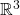, provided that the integrand is close to the area in the C1-topology. The proof is deeply inspired by posthumous notes by Almgren, devoted to the same result. Although our arguments overlap with Almgren’s, some parts are greatly simplified and rely on a nonlinear version of the planar multilinear Kakeya inequality.

Friday, 29th October 2021

### Myrto Maria Galanopoulou (Heriot-Watt University)

Abstract:

In this talk, I will present the findings of my PhD Thesis. I will examine the system of thermoelasticity endowed with polyconvex energy. I will show that we can embed the equations of polyconvex thermoviscoelasticity into an augmented, symmetrizable, hyperbolic system which possesses a convex entropy. This allows to prove many important stability results, such as convergence from the viscous problem to smooth solutions of the system of adiabatic thermoelasticity in the zero-viscosity limit. In addition, I will present a weak-strong uniqueness result in the class of entropy weak solutions and in a suitable class of measure-valued solutions, defined by means of generalized Young measures. Also, we will discuss a variational scheme for isentropic processes of adiabatic polyconvex thermoelasticity: I establish existence of minimizers which converge to a measure-valued solution that dissipates the total energy, while the scheme converges when the limiting solution is smooth.

Friday, 5th November 2021

### Gigliola Staffilani (MIT)

Abstract:

In this talk I will outline some results on the study of transfer of energy for solutions to the periodic 2D (torus domain) cubic defocusing nonlinear Schrodinger equation. In particular I will focus on the differences of the dynamics of solutions in the rational versus irrational torus. Some numerical experiments will also be presented.

(The most recent work presented is in collaboration with A. Hrabski, Y. Pan and B. Wilson.)

Friday, 19th November 2021

### Laure Saint-Raymond (IHES)

Abstract:

The dynamics of a hard sphere gas is described in the low density limit by the Boltzmann equation, at least for short times. The classical strategy  of proof, due to Lanford, fails for longer times, even close to equilibrium.In this talk, I will present a recent work with T. Bodineau, I. Gallagher and S. Simonella, where we  have  designed a new method, combining a duality and  a pruning arguments, which allows  to characterize the fluctuations around equilibrium globally in time.

Friday, 26th November 2021

### Jim Wright (University of Edinburgh)

Abstract:

In 1988 Bourgain gave a quantitative count for the number of progressions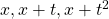in dense sets on the real line. Recently this was extended to general 3-term polynomial progressions by  X. Chen, J. Guo and X. Li. In this talk we extend these results to arbitrarily long polynomial progressions. Our methods are robust enough
to give quantitative counts for long polynomial progressions in any locally compact topological field with nontrivial topology. This is joint work with B. Krause, M. Mirek and S. Peluse.

Friday, 10th December 2021

### Jan Sbierski (University of Edinburgh)

Abstract:

The strong cosmic censorship conjecture roughly states that Einstein’s theory of general relativity is generically a deterministic theory. I will start out this talk by giving a non-technical introduction to this conjecture restricted to rotating black holes. After motivating the mathematical formulation of the strong cosmic censorship conjecture I will proceed to outline the current state of it and then conclude by presenting forthcoming work of mine showing the linear instability of the Cauchy horizon inside rotating black holes.

## Spring 2021

Friday, 22nd January 2021

### Federico Luigi Dipasquale (Università di Verona)

Abstract:

I will talk about recent results on the topology of minimizers in the Landaude Gennes (LdG) theory of nematic liquid crystals which shed light on the socalled “biaxial torus minimizers”. According to many numerical studies, these are the minimizers of the most studied LdG energy in very relevant physical conditions. Such minimizers are axially symmetric and characterized by remarkable topological properties (where the topology is to be sought in the level set of an appropriate indicator function, the signed biaxiality). However, they eluded precise mathematical description for years. In this talk, I will introduce some new key ideas which allow to study the topology of minimizers (but also of some classes of more general configurations). I will try to explain how to implement them and how this leads in a natural way to the definition of an appropriate asymptotic regime in which, in fact, topological structures are found in the biaxiality sets of minimizers. The topological results match qualitatively many features expected from the numerical simulations. We will also see that, restricting to axially symmetric configurations, we have still better accordance with simulations and much more can be said, although several interesting problems remain open. Joint work with V. Millot (Paris XIII) and A. Pisante (Sapienza).

Friday, 29th January 2021

### Franz Gmeineder (Universität Bonn)

Abstract:

By Morrey’s foundational work, quasiconvexity displays a key notion in the vectorial Calculus of Variations. A suitable generalisation that keeps track of more elaborate differential conditions is given by Fonseca & Müller’s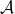-quasiconvexity. With the topic having faced numerous contributions as to lower semicontinuity, in this talk I give an overview of recent results for such problems with focus on the underlying function spaces and the (partial) regularity of minima. The talk is partially based on joint work with Sergio Conti (Bonn), Jan Kristensen (Oxford) and Lars Diening (Bielefeld).

Friday, 5th February 2021

### Eugenia Malinnikova (Stanford)

Abstract:

We consider a real-valued function on the plane for which the absolute value of the Laplacian is bounded by the absolute value of the function at each point. In other words, we look at solutions of the stationary Schrödinger equation with a bounded potential. The question discussed in the talk is how fast such function may decay at infinity. We give the answer in dimension two, in higher dimensions the corresponding problem is open. The talk is based on the joint work with A. Logunov, N. Nadirashvili, and F. Nazarov.

Friday, 12th February 2021

### Stefan Krömer (Czech Academy of Sciences)

Abstract:

Globally invertible Sobolev maps are of particular interest in models for the elastic deformation of solids, because invertibility corresponds to non-interpenetration of matter. By a result of John Ball (1981), a locally orientation preserving Sobolev map is almost everywhere globally invertible provided that its boundary values admit a homeomorphic extension. We will see that the conclusions of Ball’s theorem and related results can be reached while completely avoiding the (hard) problem of homeomorphic extension. The proof heavily relies on Brouwer’s topological degree. Among other things, the result can be applied to justify more efficient numerical approximation respecting global invertibility constraints.

Friday, 19th February 2021

### Mihalis Dafermos (Cambridge/Princeton)

Friday, 26th February 2021

### Hyunju Kwon (IAS)

Abstract:

The Euler equations describe the behavior of ideal fluids. It is well-known that smooth (spatially periodic) Euler flows conserve total kinetic energy in time. In the theory of turbulence, on the other hand, a famous conjecture of Lars Onsager asserts that kinetic energy conservation may fail when an Euler flow belongs to the Hölder spaces with Hölder exponent less than 1/3, which was proved recently by Isett. In light of these developments, I’ll discuss a stronger version of the Onsager conjecture: the existence of Hölder continuous Euler flows which locally dissipate kinetic energy.

Friday, 5th March 2021

### Jill Pipher (Brown)

Abstract:

Formulating and solving boundary value problems for divergence form real elliptic equations has been an active and productive area of research ever since the foundational work of De Giorgi – Nash – Moser established H\”older continuity of solutions when the operator coefficients are merely bounded and measurable. The solutions to such real-valued equations share some important properties with harmonic functions: maximum principles, Harnack principles, and estimates up to the boundary that enable one to solve Dirichlet problems in the classical sense of nontangential convergence. Weak solutions of complex elliptic equations and elliptic systems do not necessarily share these good properties of continuity or maximum principles. In joint work with M. Dindos, we introduced in 2017 a structural condition (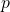-ellipticity) on divergence form complex elliptic equations that was inspired by a condition related tocontractivity due to Cialdea and Maz’ya. The-ellipticity condition was also simultaneously discovered by Carbonaro-Dragicevic, who used it to prove a bilinear embedding result. Subsequently, the condition has proven useful in the study of well-posedness of a degenerate elliptic operator associated with domains with lower-dimensional boundary. In this talk we discuss-ellipticity for complex divergence form equations, and then describe recent work, joint with J. Li and M. Dindos, extending this condition to elliptic systems. In particular, we give applications to solvability of the Dirichlet problem for the Lame systems.

Friday, 12th March 2021

### Ilaria Fragalé (Politecnico di Milano)

Abstract:

We discuss the rigidity of measurable subsets in the Euclidean space such that the Lebesgue measure of their intersection with a ball of radius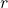, centred at any point in the essential boundary, is constant. Based on a joint work with Dorin Bucur.

Friday, 19th March 2021

### Matthew Hyde (University of Edinburgh)

Abstract:

In the nineties, Peter Jones proved the Analyst’s Travelling Salesman Theorem (TST), which gives a necessary and sufficient condition for when a set in Euclidean space can be contained in a curve of finite length. This condition is stated in terms of beta-numbers, which give a measure of how flat a set is at each location and scale. Several higher dimensional analogues of the TST have been proven since. These, along with Jones’ original result, have found many applications in fields such as complex analysis, harmonic analysis, and harmonic measure. Unlike Jones’ TST, these higher dimensional variants require additional assumption on the set. In this talk we will discuss these results in more detail and introduce a higher dimensional TST that holds for every set in Euclidean space.

Friday, 26th March 2021

### Joris Roos (University of Massachusetts Lowell & University of Edinburgh)

Abstract:

Stein and Wainger introduced an interesting class of maximal oscillatory integral operators related to Carleson’s theorem. The talk will be about joint work with Ben Krause on discrete analogues of some of these operators. These discrete analogues feature a number of substantial difficulties that are absent in the real-variable setting and involve themes from number theory and analysis.